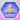Go to content
At the top right of the display, there is a place where you can specify how many marbles of each kind you wish to use. The number of marbles for each of the four colors must of course be an integer. If you put a zero as an entry, that is as if you are not putting any marbles of that color in the bag. Below, you can specify how many marbles you wish to draw at one time (1, 2 or 3 marbles). The number of marbles you draw must be greater than or equal to the total number of marbles in your 'bag'. You can decide whether order matters, in other words, would a pick of red-blue-red be the same as red-red-blue. By selecting Order Does Not Matter, the table becomes shorter for like combinations are removed. You can decide whether the marbles are replaced back in the bag after they are drawn or whether they are simply left out. You can decide how many individual trials you want to calculate with each run. By clicking Run Trials the trials are calculated. After running the trials, the data table should now include the experimental results. The first three columns indicate the order and color of the possible draw. The fourth column tells you the frequency in which that combination came up in your trials. The fifth column tells you the experimental percentage of the trials that came up with that combination and the last column tells you the theoretical percentage. You can use the scrollbar on the right to look through the trial data.From a collection of Java statistics applets from the Project
Interactive, Shodor Educational Foundation
site at Durham, North Carolina, USA, used with permission.

## Glossary

### combination

a selection of r objects from a set of n unlike objects where the order of selection is unimportant

### frequency

Of a function: the rate of repetition of a periodic function.
In statistics: the number of occurances of a value

### integer

a positive or negative whole number.

Full Glossary List

## This question appears in the following syllabi:

SyllabusModuleSectionTopicExam Year
AQA A-Level (UK - Pre-2017)S1ProbabilityExperimental-
AQA AS Maths 2017StatisticsProbabilityExperimental Probabilities-
AQA AS/A2 Maths 2017StatisticsProbabilityExperimental Probabilities-
CBSE XII (India)ProbabilityProbabilityRepeated independent (Bernoulli) trials and binomial distribution-
CBSE XI (India)Statistics and ProbabilityProbabilityRandom experiments, outcomes, sample spaces (set representation)-
CCEA A-Level (NI)S1ProbabilityExperimental-
CIE A-Level (UK)S1ProbabilityExperimental-
Edexcel A-Level (UK - Pre-2017)S1ProbabilityExperimental-
Edexcel AS Maths 2017StatisticsProbabilityExperimental Probabilities-
Edexcel AS/A2 Maths 2017StatisticsProbabilityExperimental Probabilities-
I.B. Higher Level5ProbabilityExperimental-
I.B. Standard Level5ProbabilityExperimental-
Methods (UK)M15ProbabilityExperimental-
I.B. (MSSL)3ProbabilityExperimental-
OCR A-Level (UK - Pre-2017)S1ProbabilityExperimental-
OCR AS Maths 2017StatisticsProbabilityExperimental Probabilities-
OCR MEI AS Maths 2017StatisticsProbabilityExperimental Probabilities-
OCR-MEI A-Level (UK - Pre-2017)S1ProbabilityExperimental-
Pre-Calculus (US)E4ProbabilityExperimental-
Pre-U A-Level (UK)ProbProbabilityExperimental-